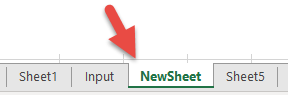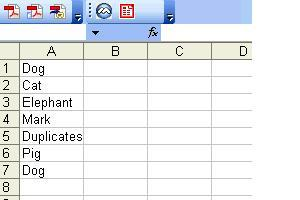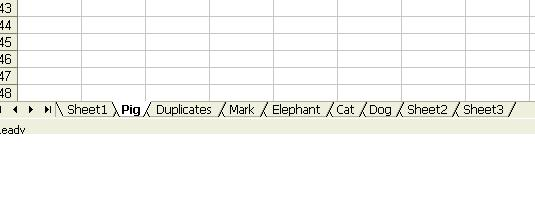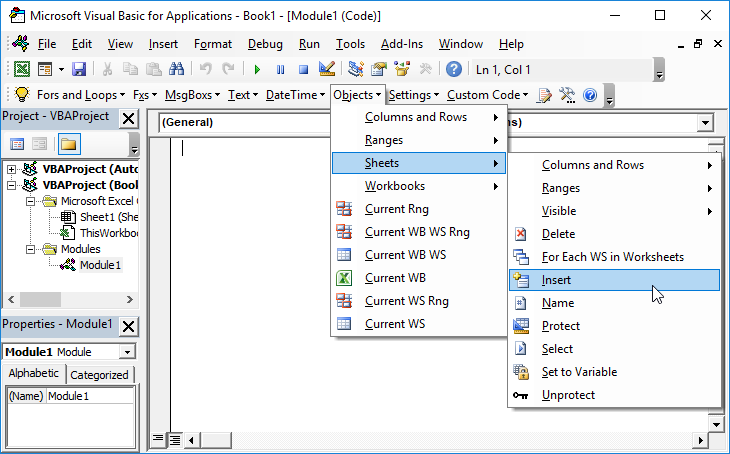# VBA routine for adding and naming sheets

This tutorial will cover how to add/insert worksheets using VBA.

This simple macro will add a sheet before the ActiveSheet:

``````Sub Add ()
End Sub``````

After you insert a worksheet, the new worksheet becomes the ActiveSheet. You can then use the ActiveSheet object to work with the new sheet (at the end of this article, we will show you how to insert a new sheet directly into a variable).

``ActiveSheet.Name = "NewSheet"``

## Add a sheet with a name

You can also define a worksheet name when creating the new sheet:

``Sheets.Add.Name = "NewSheet"``

### Create new worksheet with name from a cell

Or use a cell value to name a new worksheet:

``Sheets.Add.Name = range("a3").value``

## Add worksheet before / after another worksheet

You may also want to choose the location where the new sheet is inserted. You can use the After or Before properties to insert a worksheet in a specific location in your workbook.

### Insert worksheet after another worksheet

This code will insert the new worksheet AFTER another worksheet:

``Sheets.Add After:=Sheets("Input")``

This will insert a new worksheet AFTER another worksheet and specify the name of the worksheet:

``Sheets.Add(After:=Sheets("Input")).Name = "NewSheet"``

Note the extra parenthesis needed in the second example (the first example will generate an error if the second parenthesis is added).or Before:

``Sheets.Add(Before:=Sheets("Input")).Name = "NewSheet"``

In these examples, we explicitly name the worksheet used to determine the location of the worksheet. Often, you will want to use the index number of the worksheet instead, so you can insert the worksheet at the beginning or end of the workbook:

### Add worksheet to end of workbook

To add a worksheet to the end of the workbook:

``Sheets.Add After:=Sheets(Sheets.Count)``

### Add Sheet To Beginning of Workbook:

To add a sheet to the beginning of the workbook:

``Sheets.Add(Before:=Sheets(1)).Name = "FirstSheet"``

This code assigns the new sheet to a variable as the sheet is created:

``````Dim ws As Worksheet

From here, you can reference the new worksheet with the variable ‘ws’:

``ws.name = "VarSheet"``

## More examples of adding a worksheet

### Create sheet if it doesn’t exist yet

You might want to create a sheet only if it doesn’t already exist.

VBA Programming | Code Generator does work for you!

### Creating worksheets from a list of names

The following routine will examine the contents of a single column and create Excel worksheets in the current workbook with those names. It makes a call to another function to check if a sheet with that name already exists and if it does, the sheet will not be created.

``````Private Sub CommandButton1_Click()

Call CreateWorksheets(Sheets("Sheet2").Range("A1:a10"))

End Sub

Sub CreateWorksheets(Names_Of_Sheets As Range)
Dim Sheet_Name As String
Dim i As Integer

For i = 1 To No_Of_Sheets_to_be_Added

Sheet_Name = Names_Of_Sheets.Cells(i, 1).Value

'Only add sheet if it doesn't exist already and the name is longer than zero characters

If (Sheet_Exists(Sheet_Name) = False) And (Sheet_Name <> "") Then
End If

Next i

End Sub``````
``````Function Sheet_Exists(WorkSheet_Name As String) As Boolean
Dim Work_sheet As Worksheet

Sheet_Exists = False

For Each Work_sheet In ThisWorkbook.Worksheets

If Work_sheet.Name = WorkSheet_Name Then
Sheet_Exists = True
End If

Next

End Function``````

So if we have the following text in cells A1:A30 in Sheet 2:The following worksheets will be created:Note that although “Dog” appears twice, only one worksheet is created.